# Training Neural Networks For Stock Price Prediction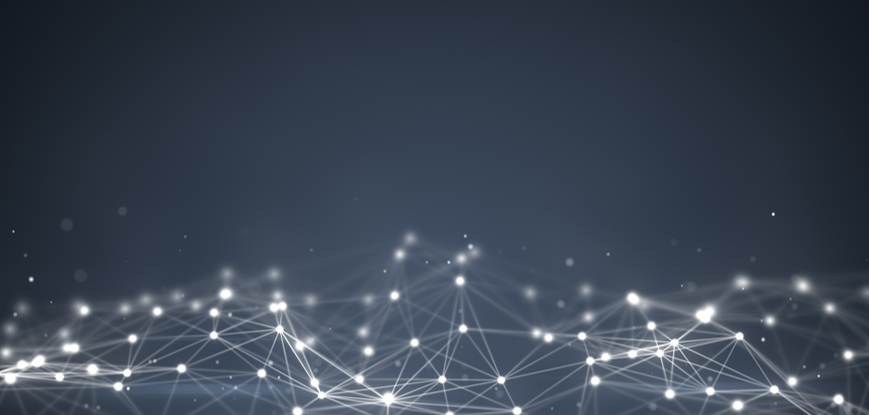### Introduction

In the previous article on “Working of Neural Networks for Stock Price Prediction”, we have understood the working of neural networks. In this article, we will look at how the model trains itself to make predictions. Once you have understood the training process, you will be ready to code your own Neural Network.

### Training the Neural Network

There are two ways to code a program for performing a specific task. One is to define all the rules required by the program to compute the result given some input to the program. The other way is to develop the framework upon which the code will learn to perform the specific task by training itself on a dataset through adjusting the result it computes to be as close to the actual results which have been observed. This process is called training the model, we will now look at how our neural network will train itself to predict stock prices.

The neural network will be given the dataset, which consists of the OHLCV data as the input and as the output, we would also give the model the Close price of the next day, this is the value that we want our model to learn to predict. The actual value of the output will be represented by ‘y’ and the predicted value will be represented by y^, y hat. The training of the model involves adjusting the weights of the variables for all the different neurons present in the neural network. This is done by minimizing the ‘Cost Function’. The cost function, as the name suggests is the cost of making a prediction using the neural network. It is a measure of how far off the predicted value, y^, is from the actual or observed value, y. There are many cost functions that are used in practice, the most popular one is computed as half of the sum of squared differences of the actual and predicted values for the training dataset.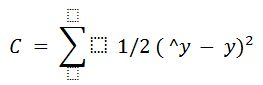The way the neural network trains itself is by first computing the cost function for the training dataset for a given set of weights for the neurons. Then it goes back and adjusts the weights, followed by computing the cost function for the training dataset based on the new weights. The process of sending the errors back to the network for adjusting the weights is called backpropagation. This is repeated several times till the cost function has been minimized. We will look at how the weights are adjusted and the cost function is minimized in more detail next.

The weights are adjusted to minimize the cost function. One way to do this is through brute force. Suppose we take 1000 values for the weights, and evaluate the cost function for these values. When we plot the graph of the cost function, we will arrive at a graph as shown below. The best value for weights would be the cost function corresponding to the minima of this graph.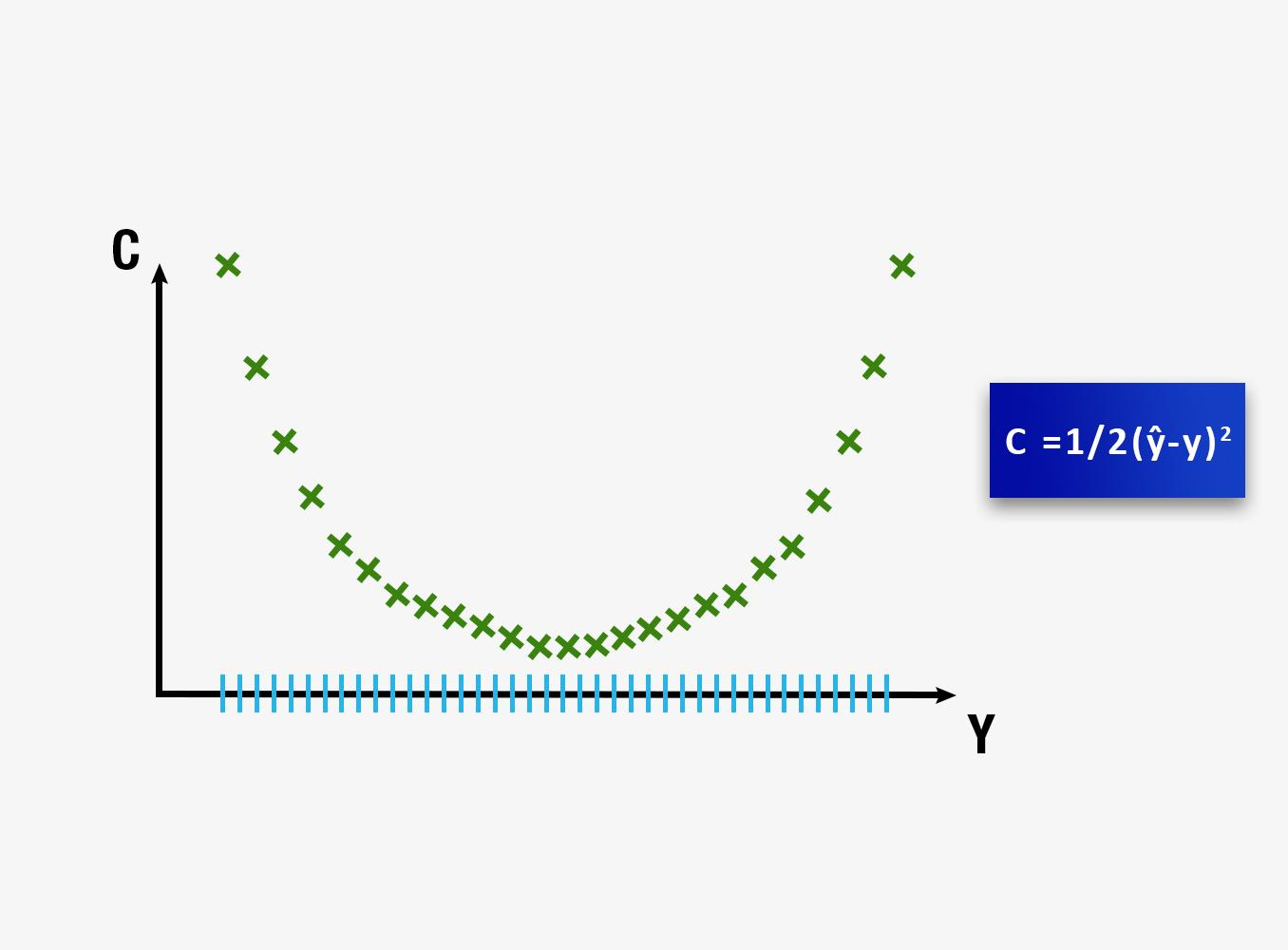This approach could be successful for a neural network involving a single weight which needs to be optimized. However, as the number of weights to be adjusted and the number of hidden layers increases, the number of computations required will increase drastically. The time it will require to train such a model will be extremely large even on the world’s fastest supercomputer. For this reason, it is essential to develop a better, faster methodology for computing the weights of the neural network. This process is called Gradient Descent.

Gradient descent involves analyzing the slope of the curve of the cost function. Based on the slope we adjust the weights, to minimize the cost function in steps rather than computing the values for all possible combinations. The visualization of Gradient descent is shown in the diagrams below. The first plot is a single value of weights and hence is two dimensional. It can be seen that the red ball moves in a zig-zag pattern to arrive at the minimum of the cost function. In the second diagram, we have to adjust two weights in order to minimize the cost function. Therefore, we can visualize it as a contour, as shown in the graph, where we are moving in the direction of the steepest slope, in order to reach the minima in the shortest duration. With this approach, we do not have to do many computations and as a result, the computations do not take very long, making the training of the model a feasible task.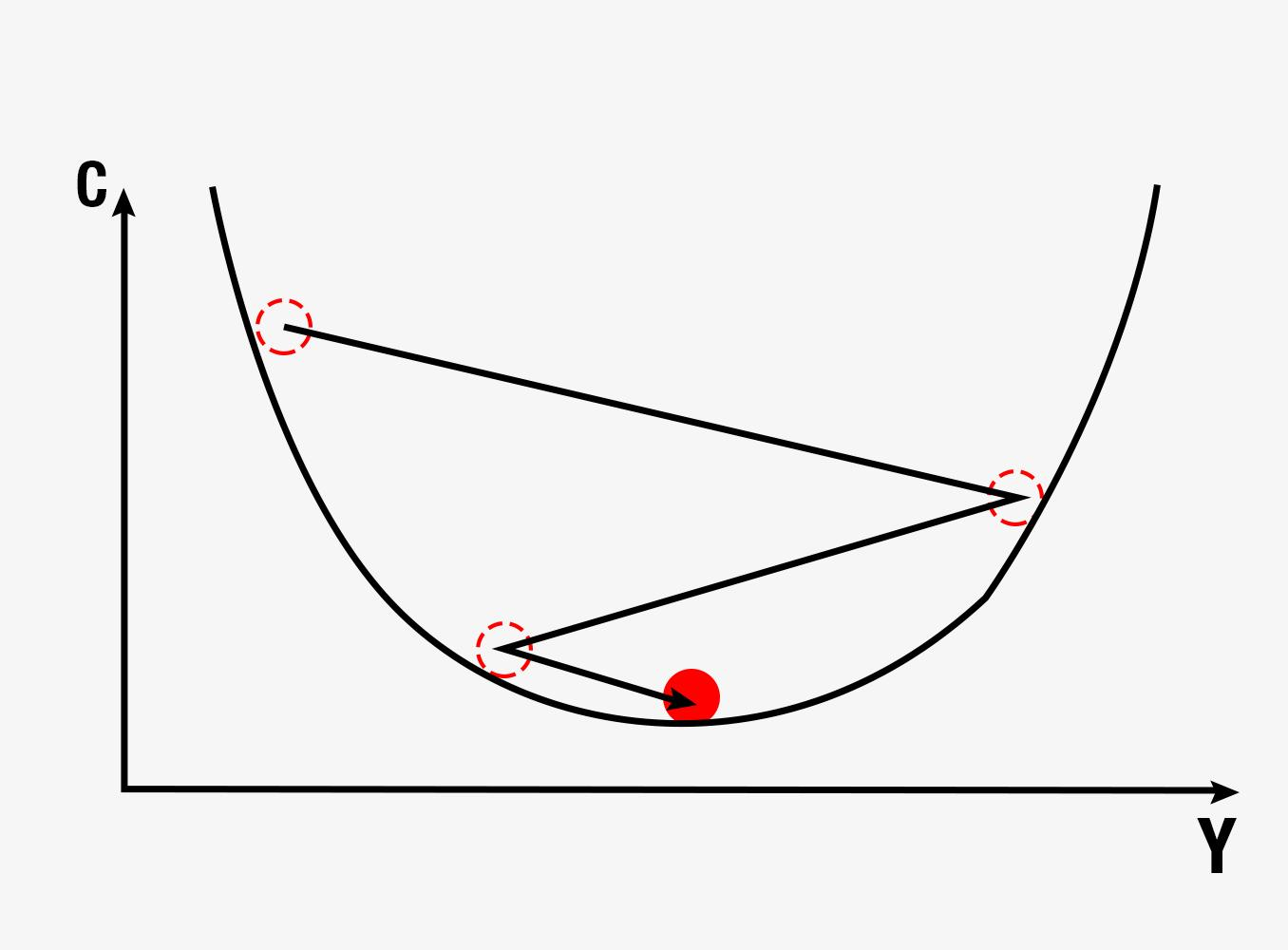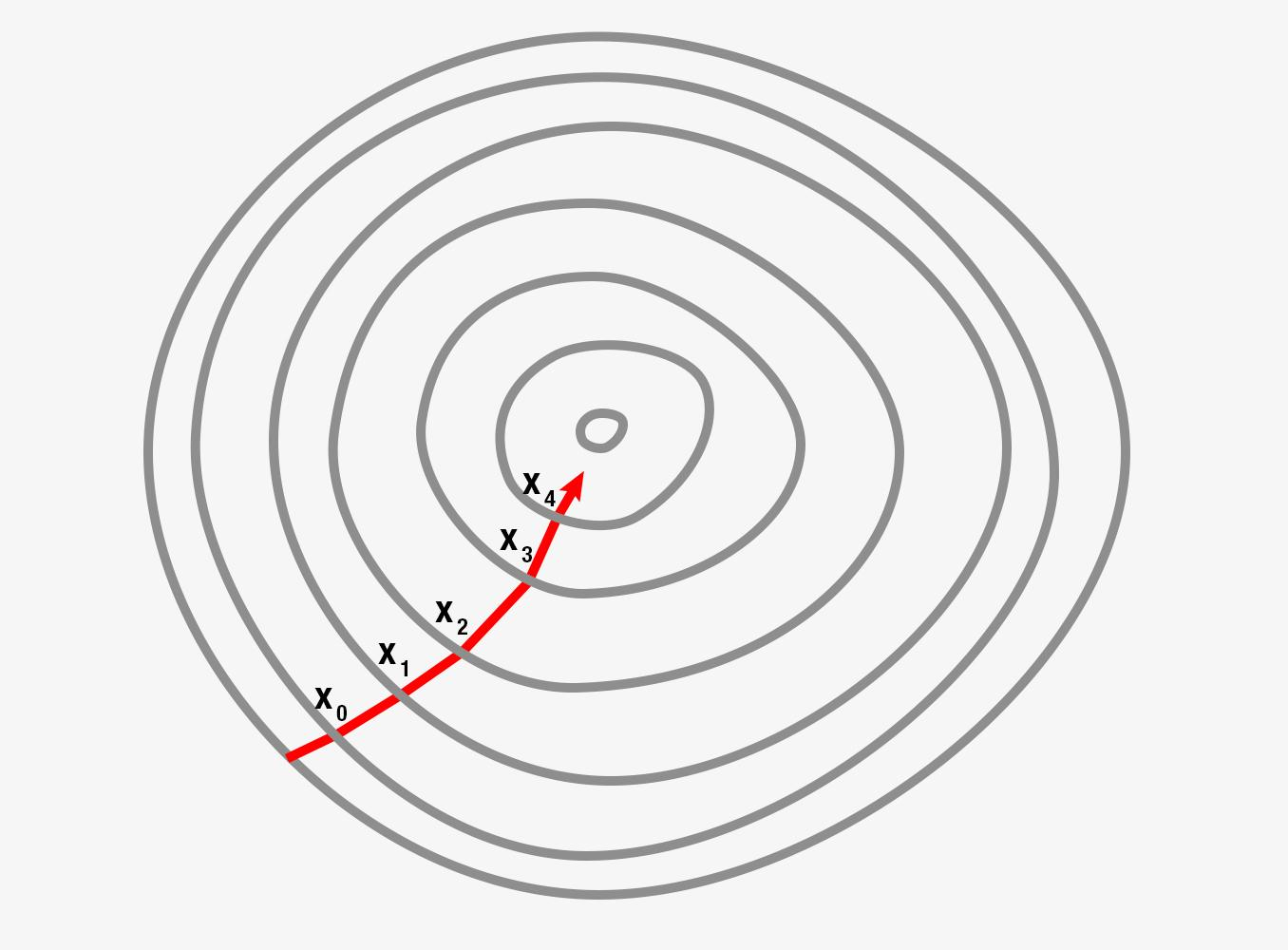### Backpropagation

Backpropagation is an advanced algorithm which enables us to update all the weights in the neural network simultaneously. This drastically reduces the complexity of the process to adjust weights. If we were not using this algorithm, we would have to adjust each weight individually by figuring out what impact that particular weight has on the error in the prediction. Let us look at the steps involved in training the neural network with Stochastic Gradient Descent:
• Initialize the weights to small numbers very close to 0 (but not 0)
• Forward propagation - the neurons are activated from left to right, by using the first data entry in our training dataset, until we arrive at the predicted result y
• Measure the error which will be generated
• Backpropagation - the error generated will be back propagated from right to left, and the weights will be adjusted according to the learning rate
• Repeat the previous three steps, forward propagation, error computation and backpropagation on the entire training dataset
• This would mark the end of the first epoch, the successive epochs will begin with the weight values of the previous epochs, we can stop this process when the cost function converges within a certain acceptable limit

### Conclusion

Now that you understand the process of building a neural network, you are ready to learn how to develop your own Artificial Neural Network to predict the movement of a stock price. In the next article on neural networks, we will build a trading strategy in python for predicting whether the stock price will move up or down during the next trading day.

### Next Step

At this moment, Artificial Intelligence and Machine Learning have already progressed enough and they can predict stock prices with a great level of accuracy. Let us take you through one such example and unveil the world of Artificial Intelligence in trading. Click here to know more.

Disclaimer:  All investments and trading in the stock market involve risk. Any decisions to place trades in the financial markets, including trading in stock or options or other financial instruments is a personal decision that should only be made after thorough research, including a personal risk and financial assessment and the engagement of professional assistance to the extent you believe necessary. The trading strategies or related information mentioned in this article is for informational purposes only.